# Mize

#### 2019-12-04

mize is a package for doing unconstrained numerical optimization. This vignette describes the use of the mize function, which takes as input a function to optimize, its gradient and a starting point and returns the optimized parameters.

First, I’ll describe the required structure of the function and gradient to optimize, then the various convergence options that apply no matter what optimization methods are being used.

In the last section, I’ll describe the methods which are available and any options that apply to them specifically.

## The fg list

If you look at stats::optim, you’ll see you pass the function to be optimized and its gradient separately. By contrast, mize asks you to bundle them up in a list, which I lazily refer to as an fg list. Here, for example, is the venerable Rosenbrock function:

rb_fg <- list(
fn = function(x) { 100 * (x - x * x) ^ 2 + (1 - x) ^ 2  },
gr = function(x) { c( -400 * x * (x - x * x) - 2 * (1 - x),
200 *        (x - x * x)) })

For minimizing a simple function, there’s not a lot of advantage to this. But for more complex optimizations, there are some advantages:

• If fn and gr require extra parameterization, you can store the extra state in a function that creates and returns the fg list, and then fn and gr can access that state via the created closure. stats::optim takes an alternative approach where extra data is passed as ... to the optim function and then internally this is passed to fn and gr when needed.
• For some optimization methods (e.g. those using a strong Wolfe line search), both fn and gr need to be evaluated for the same value of par. If there’s a lot of shared work between fn and gr, this can be quite inefficient. If you provide an optional routine called fg, which calculates the function and gradient simultaneously, this will be used by some parts of mize in preference to calling fn and gr separately, which can save a bit of time.

A version of rb_fg with an optional fg function could look like:

rb_fg <- list(
fn = function(x) { 100 * (x - x * x) ^ 2 + (1 - x) ^ 2  },
gr = function(x) { c( -400 * x * (x - x * x) - 2 * (1 - x),
200 *        (x - x * x)) },
fg = function(x) {
a <- x - x * x
b <- 1 - x
list(
fn = 100 * a ^ 2 + b ^ 2,
gr = c( -400 * x * a - 2 * b,
200 * a)
)
}
)

In the case of the Rosenbrock function, I’m not suggesting you should actually do this. See the vignette on Metric Multi-Dimensional Scaling for the sort of scenario where it could make a difference.

For the rest of this vignette, we’ll just assume you’re only providing fn and gr.

## The parameters to optimize

The parameters to optimize as passed as a numeric vector, par.

As well as using the rb_fg list we defined above to describe the Rosenbrock function, we’ll also start from the following starting point:

rb0 <- c(-1.2, 1)

## Basic options

If you don’t particularly care about how your function gets optimized, the default setting is to use the limited-memory Broyden-Fletcher-Goldfarb-Shanno (L-BFGS) optimizer, which is a good choice for pretty much anything.

You may still need to tweak some options that are independent of the method, like the maximum number of iterations to use, or other convergence criteria.

### Defaults

By default, mize will grind away on the function you give it for up to 100 iterations. But it will give up early if it senses that no further progress is being made.

The return value provides both the optimized parameter as well as some information about how much work was done to get there.

res <- mize(rb0, rb_fg)
# What were the final parameter values? (should be close to c(1, 1))
res$par #>  0.9999972 0.9999923 # What was the function value at that point (should be close to 0) res$f
#>  4.521673e-10

# How many iterations did it take?
res$iter #>  37 # How many function evaluations? res$nf
#>  49

res$ng #>  49 # Why did the optimization terminate? res$terminate
#> $what #>  "abs_tol" #> #>$val
#>  1.06609e-08

As you can see from looking at res$iter, the L-BFGS didn’t use all 100 iterations it had available. The difference between consecutive function values, res$f got sufficiently low, that the absolute tolerance criterion was passed: res$terminate$what will contain a string code that communicates what happened, while res$terminate$val provides the specific value that causes the termination, although it’s rarely interesting.

There is an entire vignette on the different convergence criteria available. The defaults should do a reasonable, albeit fairly conservative, job.

### Logging progress to console

If you want to keep track of the progress of the optimization, set the verbose option to TRUE:

res <- mize(rb0, rb_fg, grad_tol = 1e-3, ginf_tol = 1e-3, max_iter = 10,
verbose = TRUE)
#> 20:17:21 iter 0 f = 24.2 g2 = 232.9 ginf = 215.6 nf = 1 ng = 1 step = 0 alpha = 0
#> 20:17:21 iter 1 f = 19.5 g2 = 200.9 ginf = 185.6 nf = 3 ng = 3 step = 0.02169 alpha = 9.312e-05
#> 20:17:21 iter 2 f = 11.57 g2 = 135.4 ginf = 124.6 nf = 4 ng = 4 step = 0.04729 alpha = 0.3469
#> 20:17:21 iter 3 f = 4.281 g2 = 17.28 ginf = 16.27 nf = 5 ng = 5 step = 0.09809 alpha = 1
#> 20:17:21 iter 4 f = 4.144 g2 = 2.588 ginf = 2.469 nf = 6 ng = 6 step = 0.01426 alpha = 1
#> 20:17:21 iter 5 f = 4.139 g2 = 1.773 ginf = 1.626 nf = 7 ng = 7 step = 0.002565 alpha = 1
#> 20:17:21 iter 6 f = 4.132 g2 = 2.44 ginf = 2.327 nf = 8 ng = 8 step = 0.004716 alpha = 1
#> 20:17:21 iter 7 f = 4.12 g2 = 3.727 ginf = 3.033 nf = 9 ng = 9 step = 0.009086 alpha = 0.4616
#> 20:17:21 iter 8 f = 4.098 g2 = 5.427 ginf = 3.863 nf = 10 ng = 10 step = 0.01724 alpha = 0.2631
#> 20:17:21 iter 9 f = 2.569 g2 = 8.248 ginf = 7.431 nf = 16 ng = 16 step = 0.8311 alpha = 4.743
#> 20:17:21 iter 10 f = 2.553 g2 = 11.67 ginf = 9.996 nf = 18 ng = 18 step = 0.04657 alpha = 0.05068

As you can see, information about the state of the optimization is logged to the console every time the convergence criteria are checked. The values logged are:

• f - The current value of the function.
• g2 - The current value of the gradient 2-norm (if you set a non-NULL grad_tol).
• ginf - The current value of the gradient infinity norm (if you set a non-NULL ginf_tol).
• nf - The total number of function evaluations so far.
• ng - The total number of gradient evaluations so far.
• step - The magnitude of the change between the previous version of par and the current value.

Note that values are also provided for iteration 0, which represents the state of par at its starting point. Because this requires a function evaluation that might otherwise not be needed, you may get results where nf and ng are one larger than you’d otherwise expect if verbose was FALSE.

Logging every iteration might be more output than you want. You can also set the log_every parameter to make logging slightly less noisy:

res <- mize(rb0, rb_fg, grad_tol = 1e-3, verbose = TRUE, log_every = 10)
#> 20:17:21 iter 0 f = 24.2 g2 = 232.9 nf = 1 ng = 1 step = 0 alpha = 0
#> 20:17:21 iter 10 f = 2.553 g2 = 11.67 nf = 18 ng = 18 step = 0.04657 alpha = 0.05068
#> 20:17:21 iter 20 f = 0.5142 g2 = 3.001 nf = 31 ng = 31 step = 0.1319 alpha = 1
#> 20:17:21 iter 30 f = 0.009862 g2 = 3.333 nf = 42 ng = 42 step = 0.03554 alpha = 0.1706
#> 20:17:21 iter 37 f = 4.522e-10 g2 = 0.0009379 nf = 49 ng = 49 step = 0.0002131 alpha = 1

On the assumption that you probably want to see information about the last iteration, this is also reported, even if it wouldn’t normally be reported according to the setting for log_every.

### Storing progress

You may wish to do more than stare at the progress numbers logged to the console during optimization when verbose = TRUE. If you would like access to these values after optimization, set the store_progress parameter to true, and a data frame called progress will be part of the return value:

res <- mize(rb0, rb_fg, store_progress = TRUE, log_every = 10)
res$progress #> f nf ng step alpha #> 0 2.420000e+01 1 0 0.0000000000 0.00000000 #> 10 2.552598e+00 18 18 0.0465699833 0.05067737 #> 20 5.142086e-01 31 31 0.1319347626 1.00000000 #> 30 9.862134e-03 42 42 0.0355364981 0.17057755 #> 37 4.521673e-10 49 49 0.0002130984 1.00000000 As can be seen, store_progress will also obey the log_every parameter and not store every iteration of convergence information if requested. ## Optimization Methods The available optimization methods are listed below. I won’t be describing the algorithms behind them in any depth. For that, you should look in the book by Nocedal and Wright. Many of these methods can be thought of as coming up with a direction to move in, but with the distance to travel in that direction as being someone else’s problem, i.e. a line search method needs to also be used to determine the step size. We’ll discuss the available step size methods separately. ### Steepest Descent ("SD") The good news is that you always get some improvement with steepest descent. The bad news is everything else: it often takes a long time to make any progress, unless you are very clever with the step size. It is however, the basis for most other gradient descent techniques, and many of them will revert back to this direction, at least temporarily, if things start going wrong. res <- mize(rb0, rb_fg, max_iter = 10, method = "SD") ### Broyden-Fletcher-Goldfarb-Shanno ("BFGS") The BFGS method is a quasi-Newton method: it constructs an approximation to the inverse of the Hessian. This saves on the annoyance of working out what the second derivatives are for your problem, as well as on the time it takes to calculate them on every iteration, but not on the storage cost, which is O(N^2). It’s therefore not appropriate for large problems. res <- mize(rb0, rb_fg, max_iter = 10, method = "BFGS") By default, after the first iteration, the inverse Hessian approximation is scaled to make it more similar to the real inverse Hessian (in terms of eigenvalues). If you don’t want to do this, set the scale_hess parameter to FALSE: res <- mize(rb0, rb_fg, max_iter = 10, method = "BFGS", scale_hess = FALSE) ### Limited-Memory BFGS ("L-BFGS") The L-BFGS method builds the inverse Hessian approximation implicitly, yielding something close to the BFGS direction without ever directly creating a matrix. This makes it suitable for larger problems. The closeness to the BFGS result is determined by the size of memory parameter, which determines the number of previous updates that are used to create the direction for the next iteration. The higher this value, the closer to the BFGS result will be obtained, but the more storage is required. In practice, the recommended memory size is between 3 and 7. The default is 5. res <- mize(rb0, rb_fg, max_iter = 10, method = "L-BFGS", memory = 7) The L-BFGS method also applies a similar Hessian scaling method as with the BFGS method, but does so at every iteration. If you don’t want it for some reason, you can also set scale_hess to FALSE: res <- mize(rb0, rb_fg, max_iter = 10, method = "L-BFGS", scale_hess = FALSE) ### Conjugate Gradient ("CG") When someone is pulling a decent default optimization off the shelf, if they’re not reaching for L-BFGS, they’re probably going for a conjugate gradient method. In their favor, they require less storage than the L-BFGS. However, they tend to be a lot more sensitive to poor scaling of the function (i.e. when changing different parameters causes very different changes to the objective function) and so need tighter line search criteria to make progress. There are in fact multiple different methods out there under the name ‘conjugate gradient’, which differ in how the direction is determined from the current and previous gradient and the previous direction. The default method in mize is the “PR+” method (sometimes referred to as “PRP+”), which refers to its inventors, Polak and Ribiere (the third “P” is Polyak who independently discovered it). The “+” indicates that a restart to steepest descent is included under certain conditions, which prevents the possibility of convergence failing. res <- mize(rb0, rb_fg, max_iter = 10, method = "CG") Other CG update methods are available via the cg_update parameter. Methods which seem competitive or even superior to the "PR+" update is the "HS+" method (named after Hestenes and Stiefel) and the "HZ+" update (named after Hager and Zhang, and part of the CG_DESCENT optimizer): res <- mize(rb0, rb_fg, max_iter = 10, method = "CG", cg_update = "HZ+") ### Nesterov Accelerated Gradient ("NAG") The Nesterov Accelerated Gradient method consists of doing a steepest descent step immediately followed by a step that resembles momentum (see below), but with the previous gradient term replaced with the current gradient. res <- mize(rb0, rb_fg, max_iter = 10, method = "NAG") Be aware that the convergence of NAG is not guaranteed to be monotone, that is you may (and probably will) see the function value increase between iterations. Getting the optimum convergence rates guaranteed by theory requires you to be able to estimate how strong the convexity of the function you are minimizing is. To see this in action, let’s increase the iteration count to 100 and plot the progress of the function: res <- mize(rb0, rb_fg, max_iter = 100, method = "NAG", store_progress = TRUE) plot(res$progress$nf, log(res$progress$f), type = "l")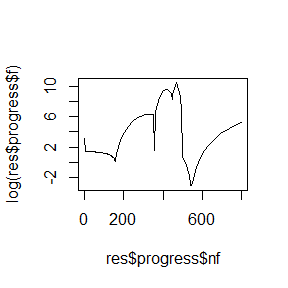res$f
#>  0.04420106

Yikes. If you let this optimization continue, it does eventually settle down, but it’s a real test of patience. Clearly, in this case, there’s probably a better estimate of the strong convexity parameter.

You can control the strong convexity estimate with the nest_q parameter, which is inversely related to the amount of momentum that is applied. It should take a value between 0 (you think your function is strongly convex) and 1 (method becomes steepest descent). The default is zero, and this is in fact the assumed value in most applications and papers.

Let’s try turning down the momentum a little bit and repeat:

resq <- mize(rb0, rb_fg, max_iter = 100, method = "NAG", nest_q = 0.001,
store_progress = TRUE)
plot(res$progress$nf, log(res$progress$f), type = "l",
ylim = range(log(res$progress$f), log(resq$progress$f)))
lines(resq$progress$nf, log(resq$progress$f), col = "red")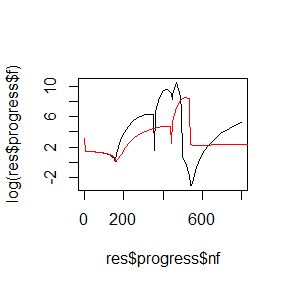resq$f #>  1.147505 The red line is the result with nest_q = 0.001. That’s better. Unfortunately, I have no advice on how you should go about estimating the right value for nest_q. However, see the section on Adaptive Restart for ways to ameliorate the problem. To get the theoretical guarantees about convergence, you actually need to follow a fairly strict formula about what the step size should be (it’s related to the reciprocal of the Lipschitz constant of the function), and Sutskever notes that for non-convex functions (with sparse stochastic gradient) the theoretically optimal momentum and step size values are too aggressive. Alas, the best advice (at least for deep learning applications) given was “manual tuning”. There are connections between NAG and classical momentum, and there is some evidence (at least in deep learning) that replacing classical momentum with the NAG method, but using the same momentum schedule (see below) can improve results. I have written more (much more) on the subject here. ### Classical Momentum ("MOM") Momentum methods use steepest descent and then add a fraction of the previous iteration’s direction. Often the effectiveness of this approach is likened to inertia and has the effect of damping the oscillation in direction that slows the progress of steepest descent with poorly-scaled functions. Originally popular for training neural networks, the momentum value is often a constant and set to a fairly high value, like 0.9. You can set a constant momentum by setting the mom_schedule parameter: res <- mize(rb0, rb_fg, max_iter = 10, method = "MOM", mom_schedule = 0.9) Momentum-based methods share the same issues as Nesterov Accelerated Gradient: convergence need not be monotone. The plot below demonstrates: res <- mize(rb0, rb_fg, max_iter = 100, method = "MOM", mom_schedule = 0.9, store_progress = TRUE) plot(res$progress$nf, log(res$progress$f), type = "l")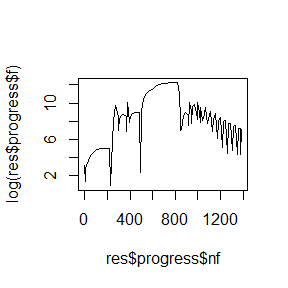res$f
#>  2.414765

To be fair, there is little reason to believe that the sort of settings used for neural network training would be effective for minimizing the Rosenbrock function.

Non-constant momentum schedules are also available, by passing a string to mom_schedule. These include:

• A switch function, that steps the momentum from one value (mom_init), to another (mom_final) at a specified iteration (mom_switch_iter):
# Switch from a momentum of 0.4 to 0.8 at iteration 5
res <- mize(rb0, rb_fg, max_iter = 10, method = "MOM", mom_schedule = "switch",
mom_init = 0.4, mom_final = 0.8, mom_switch_iter = 5)
• A ramp function, the linearly increases the momentum from mom_init to mom_final over max_iter iterations:
res <- mize(rb0, rb_fg, max_iter = 10, method = "MOM", mom_schedule = "ramp",
mom_init = 0.4, mom_final = 0.8)
• The schedule used in NAG can be used here by asking for a "nsconvex" schedule.
res <- mize(rb0, rb_fg, max_iter = 10, method = "MOM", mom_schedule = "nsconvex")
• The nest_q parameter can also be used here as it can be with NAG:
res <- mize(rb0, rb_fg, max_iter = 10, method = "MOM", mom_schedule = "nsconvex",
nest_q = 0.001)

If none of this meets your needs, you can pass a function that takes the current iteration number and the maximum number of iterations as input and returns a scalar, which will be used as the momentum value, although the value will be clamped between 0 and 1.

For example, you could create a momentum schedule that randomly picks a value between 0 and 1:

mom_fn <- function(iter, max_iter) {
runif(n = 1, min = 0, max = 1)
}
res <- mize(rb0, rb_fg, max_iter = 10, method = "MOM", mom_schedule = mom_fn)

I’m not saying it’s a good idea.

### Simplified Nesterov Momentum

Interest in NAG in the neural network community (specifically deep learning), was sparked by papers from Sutskever and co-workers and Bengio and co-workers who showed that it could be related to classical momentum in a way that made it easy to implement if you already had a working classical momentum implementation, and more importantly, that it seemed to give improvements over classical momentum.

As mize already offers NAG by setting method = "NAG", being able to use it in a different form related to classical momentum may seem superfluous. But in fact, the use of what’s often called “NAG” in deep learning is slightly more general (and as far as I’m aware, has no convergence theory to go with it, so improvements are not guaranteed). It’s probably better to refer to it as (simplified) Nesterov momentum.

At any rate, the key observation of Sutskever was that NAG could be considered a momentum method where the momentum stage occurs before the gradient descent stage. In this formulation, you can have whatever momentum schedule you like, and the hope is that the gradient descent stage can more easily correct if the momentum schedule is too aggressive because it gets to “see” the result of the momentum update and hence the descent occurs from a different location.

To get Nesterov momentum, you need only add a mom_type = "nesterov" parameter to a momentum optimizer:

res <- mize(rb0, rb_fg, max_iter = 10, method = "MOM", mom_schedule = 0.9,
mom_type = "nesterov")

I know you’re itching to see whether Nesterov momentum can calm down the bad case of non-monotone convergence we got with a large classical momentum value on the Rosenbrock function:

resc <- mize(rb0, rb_fg, max_iter = 100, method = "MOM", mom_schedule = 0.9,
store_progress = TRUE)
resn <- mize(rb0, rb_fg, max_iter = 100, method = "MOM", mom_schedule = 0.9,
mom_type = "nesterov",
store_progress = TRUE)
# Best f found for Nesterov momentum
resn$f #>  1.069263 # Best f found for classical momentum resc$f
#>  2.414765
plot(resc$progress$nf, log(resc$progress$f), type = "l",
ylim = range(log(resc$progress$f), log(resn$progress$f)))
lines(resn$progress$nf, log(resn$progress$f), col = "red")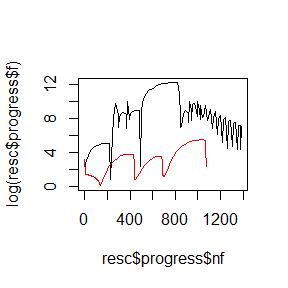The Nesterov momentum is in red. And yes, it’s a lot smoother. On the other hand, you can see that the cost function increases and then levels off. Clearly, some tweaking of the correct momentum schedule to use is needed.

Sutskever also came up with a simplified approximation to the momentum schedule used in NAG. It doesn’t have a big effect on the run time, only applies for the default nest_q of 0, and at early iterations the momentum is much larger. To use it, set the nest_convex_approx = TRUE when using the mom_schedule = "nesterov":

res <- mize(rb0, rb_fg, max_iter = 10, method = "MOM",
mom_schedule = "nsconvex", nest_convex_approx = TRUE,
mom_type = "nesterov")

## Line Searches

All the above methods need a line search to find out how far to move in the gradient descent (i.e. non-momentum) part.

### Other Line Searches

The Wolfe line search is your best bet, but there are some alternatives (of somewhat dubious value).

You can set a constant value for the step size. But note that the total step size is actually determined by the product of the step size and the length of the gradient vector, so you may want to set norm_direction too:

res <- mize(rb0, rb_fg, max_iter = 10, method = "SD", line_search = "constant",
norm_direction = TRUE, step0 = 0.01)

There is also a backtracking line search, which repeatedly reduces the step size starting from step0, until the sufficient decrease condition (also known as the Armijo condition) of the Wolfe line search conditions is fulfilled. This is controlled by c1, the larger the value, the larger the decrease in the function value is needed for a step size to be accepted. By default, the cubic interpolation method from minFunc is used to find the step size.

res <- mize(rb0, rb_fg, max_iter = 10, line_search = "backtracking", step0 = 1,
c1 = 0.1)

You will often see a simple fixed step size reduction used in combination with this line search, mainly due to ease of implementation. Cubic interpolation is probably always preferable, but if you want to use a constant step size reduction, provide a non-NULL value to step_down. The step size will be multiplied by this value so it should be a value between zero and one. Set it to 0.5, for example, to halve the step size while backtracking:

res <- mize(rb0, rb_fg, max_iter = 10, line_search = "backtracking",
step0 = 1, c1 = 0.1, step_down = 0.5)

A similar approach is the "bold driver", which also backtracks, but starts from the step size found for the last iteration, increased by a factor step_up.

# increase step size by 10%, but reduce by 50%
res <- mize(rb0, rb_fg, max_iter = 10, line_search = "bold",
step0 = 1, step_down = 0.5, step_up = 1.1)

Unlike backtracking line search, the bold driver accepts any function decrease, and does not use c1.

So far, the method and line_search arguments can be freely mixed and matched. However, some methods choose both the direction and the step size directly. The Delta-Bar-Delta method has its roots in neural network research but was also used in the currently popular t-Distributed Stochastic Neighbor Embedding data visualization method.

The way DBD works is to consider each component of the gradient and a weighted average of previous iterates gradients. If the sign has changed for that component, that suggests the minimum has been skipped, so the step size (where each component is initialized with value step0) is reduced (by a factor step_down) in that direction. Otherwise the step size is increased (by a factor step_up). The weighting of the average of previous gradients is controlled by dbd_theta, which varies between 0 and 1.

The same line search parameters that control the bold driver method can be used in DBD:

res <- mize(rb0, rb_fg, max_iter = 10, method = "DBD",
step0 = "rasmussen", step_down = 0.5, step_up = 1.1,
dbd_weight = 0.5)

One extra parameter is available: step_up_fn. In the original DBD paper, when the new step size is to be increased for a component, it is done by adding the value of step_up to the current value of the step size. Janet and co-workers noted that when the step sizes are small compared to the value of step_up this can lead to far too large a step size increase. Instead, they suggest multiplying by step_up so that a percentage increase in the step size is achieved. This is the default behavior in mize, but the t-SNE optimization method uses the addition method. To get this behavior, set the step_up_fun value to "+":

res <- mize(rb0, rb_fg, max_iter = 10, method = "DBD",
step0 = "rasmussen", step_down = 0.8, step_up = 0.2,
step_up_fun = "+")

The DBD method can be used with momentum. If it is, then the dbd_weight parameter is ignored and instead of storing its own history of weighted gradients, the update vector stored by the momentum routines is used instead. This is not mentioned in the original paper, but is used in the t-SNE implementation.

The DBD method is interesting in that it uses no function evaluations at all in its optimization. It doesn’t even avail itself of the values of any of the gradient components, only their signs. However, as a result, it’s really, really easy for DBD to diverge horribly and quickly. You will therefore want to ensure that you are checking the convergence. This is one of the methods where using the grad_tol or ginf_tol is a better idea than abs_tol and rel_tol, because it provides some check on divergence without calculating the function ever iteration only for convergence checking purposes.

# DBD with rel_tol and abs_tol is explicitly set
res <- mize(rb0, rb_fg, max_iter = 10, method = "DBD",
step0 = "rasmussen", step_down = 0.8, step_up = 0.2,
step_up_fun = "+", rel_tol = 1e-8, abs_tol = 1e-8)
# 10 gradient calculations as expected
res$ng #>  10 # But 10 function calculations too, only used in the tolerance check res$nf
#>  10

# Turn off the rel_tol and abs_tol and let max_iter handle termination
res <- mize(rb0, rb_fg, max_iter = 10, method = "DBD",
step0 = "rasmussen", step_down = 0.8, step_up = 0.2,
step_up_fun = "+", rel_tol = NULL, abs_tol = NULL,
res$ng #>  11 # Only one function evalation needed (to calculate res$f)
res$nf #>  1 Using grad_tol results in one extra gradient calculation (because it using gradients for the convergence check), but only one function evaluation. The more iterations this runs for, the better trade off this is, although in the event of a diverging optimization, the “best” par that is returned may not be as good as would have been returned if we were also using function evaluations. ## Adaptive Restart O’Donoghue and Candes described a restart scheme for NAG as a cure for the non-monotonic convergence. They suggest using either a function-based check (did the function value after the gradient descent stage decrease between iterations?) or a gradient-based check (is the momentum direction a descent direction?). If the check fails, then the momentum is restarted: the previous update vector is discarded and the momentum set back to zero. mize allows these checks to be used to restart any momentum scheme, not just NAG. The only change is that the function-based check is carried out at the beginning and end of each iteration, rather than at the end of the gradient descent stage. Restarting is applied using the restart argument, supplying either fn or gr for function-based and gradient-based restarting, respectively: resc <- mize(rb0, rb_fg, max_iter = 100, method = "MOM", mom_schedule = 0.9, store_progress = TRUE) resf <- mize(rb0, rb_fg, max_iter = 100, method = "MOM", mom_schedule = 0.9, store_progress = TRUE, restart = "fn") resg <- mize(rb0, rb_fg, max_iter = 100, method = "MOM", mom_schedule = 0.9, store_progress = TRUE, restart = "gr") plot(resc$progress$nf, log(resc$progress$f), type = "l", ylim = range(log(resc$progress$f), log(resf$progress$f), log(resg$progress$f))) lines(resf$progress$nf, log(resf$progress$f), col = "red") lines(resg$progress$nf, log(resg$progress$f), col = "blue")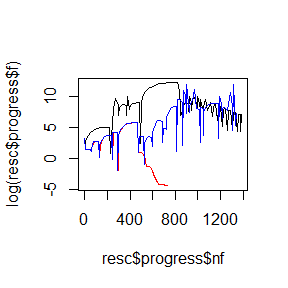Adaptive restart (red and blue lines) certainly seems to help here, although with either gradient or function-based restart seeming equally effective. The only downside to adaptive restart is that you may want to choose the type of restart to not require more overhead of function and gradient calls than necessary. In practice, the gradient-based restart is economical because in most cases, the gradient calculated at the end of the iteration can be re-used for the gradient descent at the start of the next iteration. The one exception here is when using nesterov momentum, where the gradients aren’t calculated in the right location to be of use. In that case, you are better off using function-based restarting, although if you aren’t checking convergence with a function calculation or using a line search method that makes use of the function at the starting point, you will obviously incur the overhead of the function call. This is one reason to favour using the NAG method directly rather than emulating it with Nesterov momentum. There is also a restart_wait parameter. This determines how many iterations to wait between attempted restarts. By default, using the value from the paper of Su and co-workers, we wait 10 iterations before allowing another restart. Making the wait time too short may lead to premature convergence: resfw <- mize(rb0, rb_fg, max_iter = 100, method = "MOM", mom_schedule = 0.9, store_progress = TRUE, restart = "fn", restart_wait = 1) resgw <- mize(rb0, rb_fg, max_iter = 100, method = "MOM", mom_schedule = 0.9, store_progress = TRUE, restart = "gr", restart_wait = 1) plot(resc$progress$nf, log(resc$progress$f), type = "l", ylim = range(log(resc$progress$f), log(resf$progress$f), log(resg$progress$f), log(resfw$progress$f), log(resgw$progress$f))) lines(resf$progress$nf, log(resf$progress$f), col = "red") lines(resfw$progress$nf, log(resfw$progress$f), col = "blue") lines(resgw$progress$nf, log(resgw$progress\$f), col = "orange")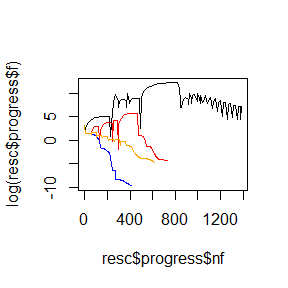There is also a "speed" restart type, related to a restart strategy introduced by Su and co-workers, which restarts if the update vector doesn’t increase in length (as measured by Euclidean 2-norm) between iterations. This seems less performant in their experiments than gradient-based restarting, but might provide more stability under some circumstances.